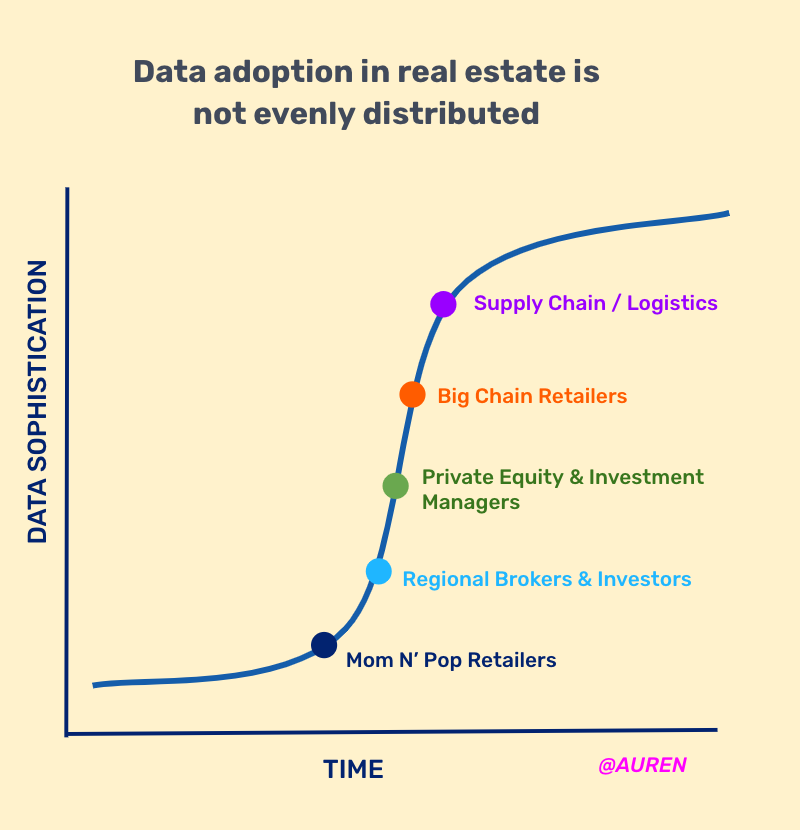Blog home

# Demographic Profiles with Rigorous Statistics Using SafeGraph Patterns Data

November 14, 2019
by
Ryan Fox Squire

## How to separate the signal from the noise?

In Part 3 we showed how to do full-fledged multi-dimensional demographic analysis with ease. We can produce results like those shown below in Figure 1. If you want to reproduce these analyses without the pedagogy, use the Workbook for Demographic Analysis Jupyter Co-Lab Notebook.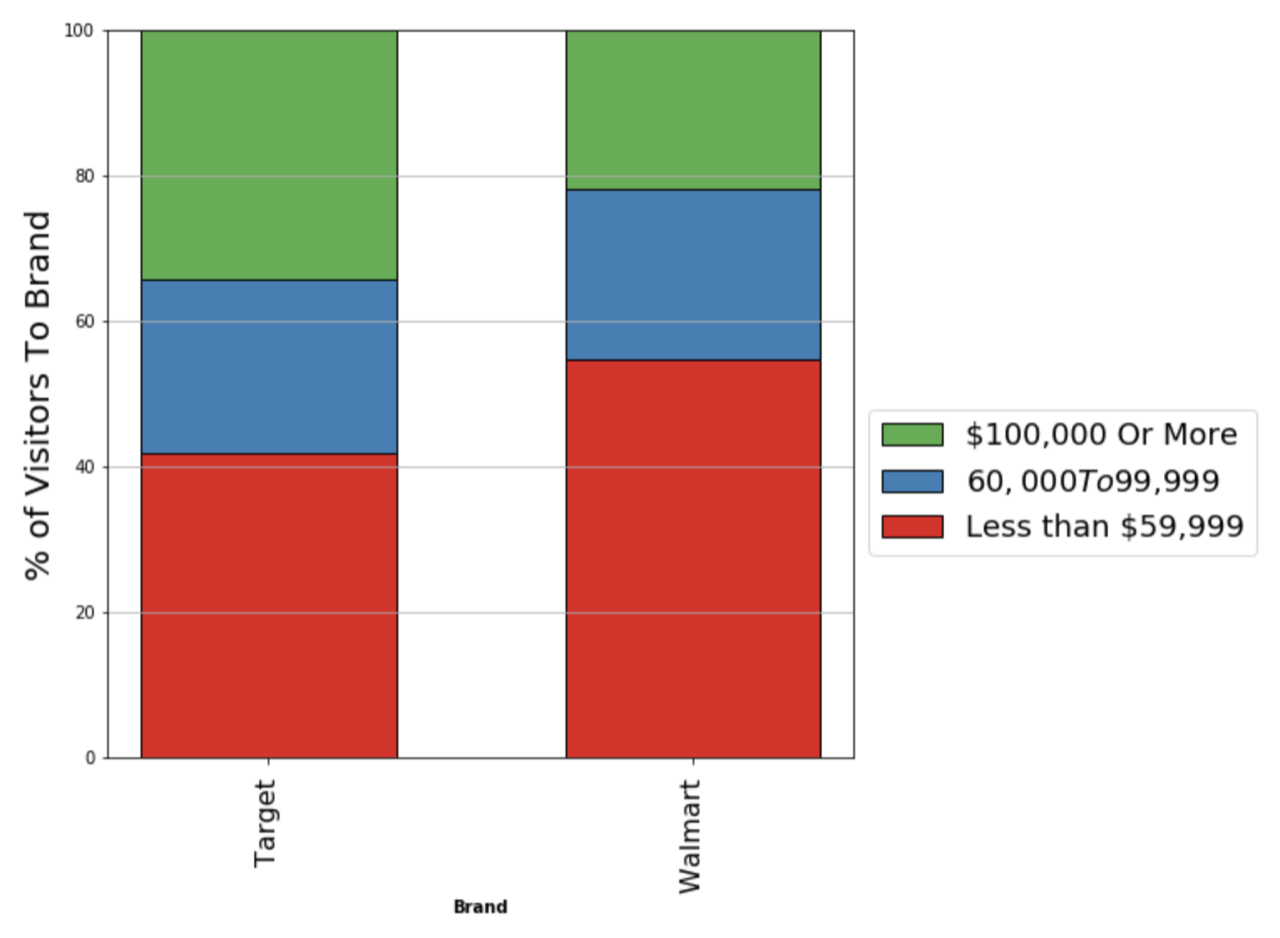Figure 1: Customer demographics for household income for Target vs Walmart, aggregated over all locations nationally. Want to make this chart yourself? See Part 3.

The data in Figure 1 suggests that Target customers are more likely to have higher household incomes (based on where they live) compared to customers of Walmart. But how do we know that these are statistically meaningful differences and not just due to random chance from a noisy dataset?

## As sample sizes get smaller, statistical methodology becomes more important. Example: Single-location demographic profiles.

To motivate the need to quantify uncertainty, let’s imagine a new question. Instead of analyzing Target and Walmart nation-wide (Figure 1), we want to analyze a single Target location and a single Walmart location, both located in the same neighborhood.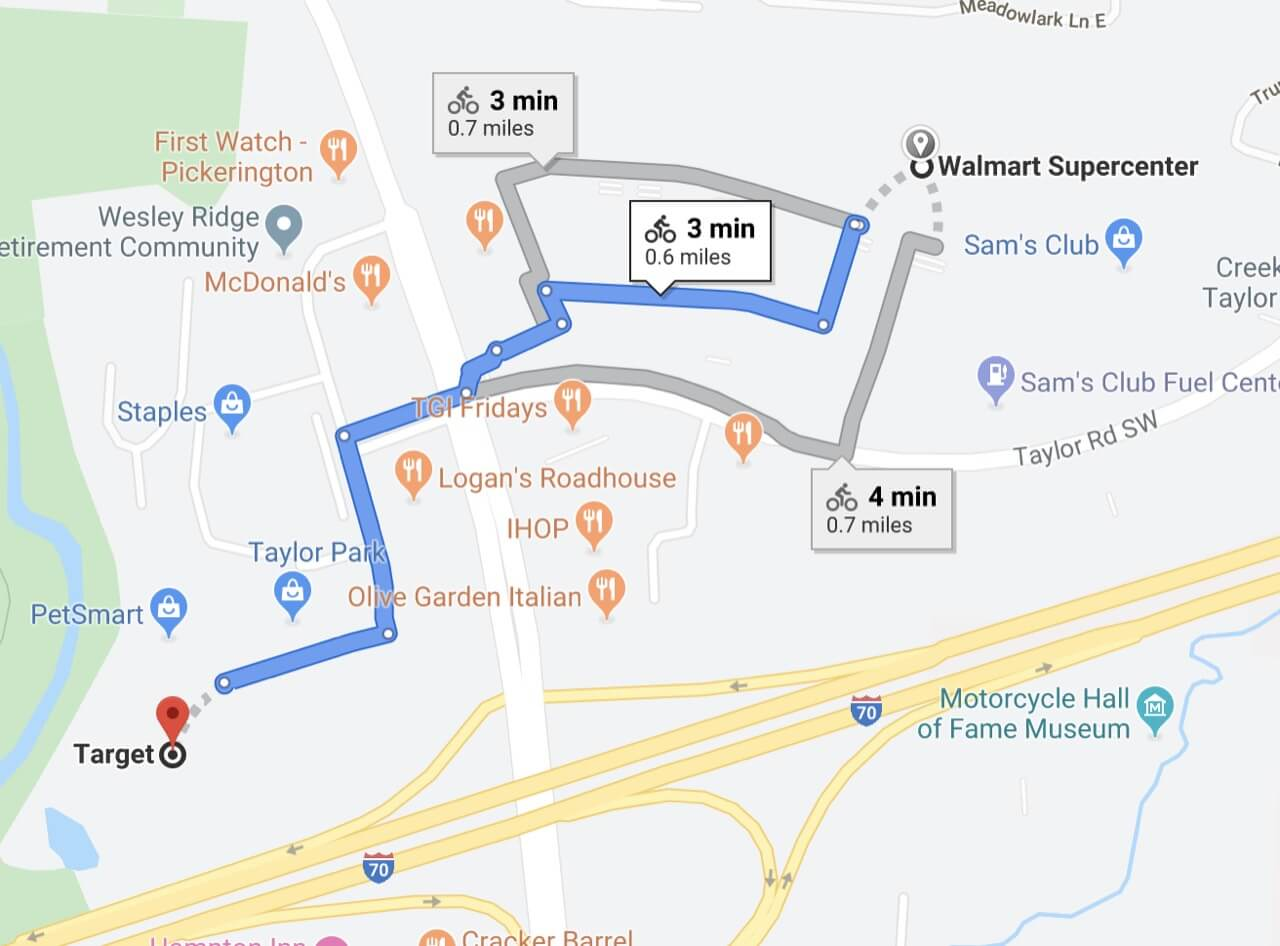Figure 2. safegraph_place_ids sg:08a06860e8ec49b0b4cc9d74d8042b06 and sg:ea46efc2e7744380b1fb1e84491411ed are a Target and a Walmart located less than 1 mile apart in Reynoldsburg, OH.

Here is an example in Reynoldsburg, OH of a Target and a Walmart that are ~ 0.5 miles apart. They are equally geographically accessible to all the demographic segments of this community. Given their geographic proximity, do the two stores have different customer demographics?

We can analyze these two locations using the variable safegraph_place_id_whitelist.

CODE: https://gist.github.com/ryanfoxsquire/be349b8e90cfc0df2f4308fe7686ea01.js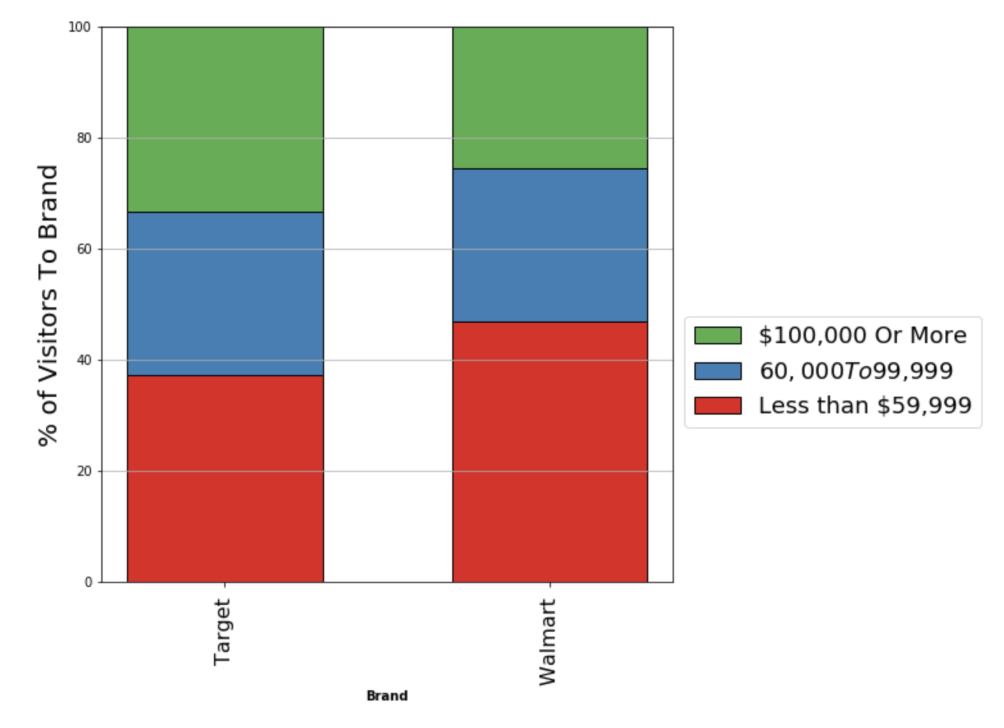Figure 3: Customer Demographics for a single location of Target vs a single location of Walmart located in the same neighborhood (see POI and locations in Figure 2).

Figure 3 summarizes the demographics of customers to a single Target location (left) and a single Walmart location (right). These results suggest similar differences as to what was found nationally (see Figure 1) but have been built from a much smaller sample size (only a single location for each brand). Are these differences real, or could these results just be noise from random chance?

The purpose of statistics is to quantify our uncertainty. Let’s use statistics to decide whether the differences between this Target and Walmart are real or statistical noise.

These statistics are available to you via the show_errors flag.

CODE: https://gist.github.com/ryanfoxsquire/36112fcdfa557e5d358d1700f3f748fa.js

This is identical to the previous code block except for the show_error=True variable in make_demographics_chart(). The above code produces the chart shown in Figure 4.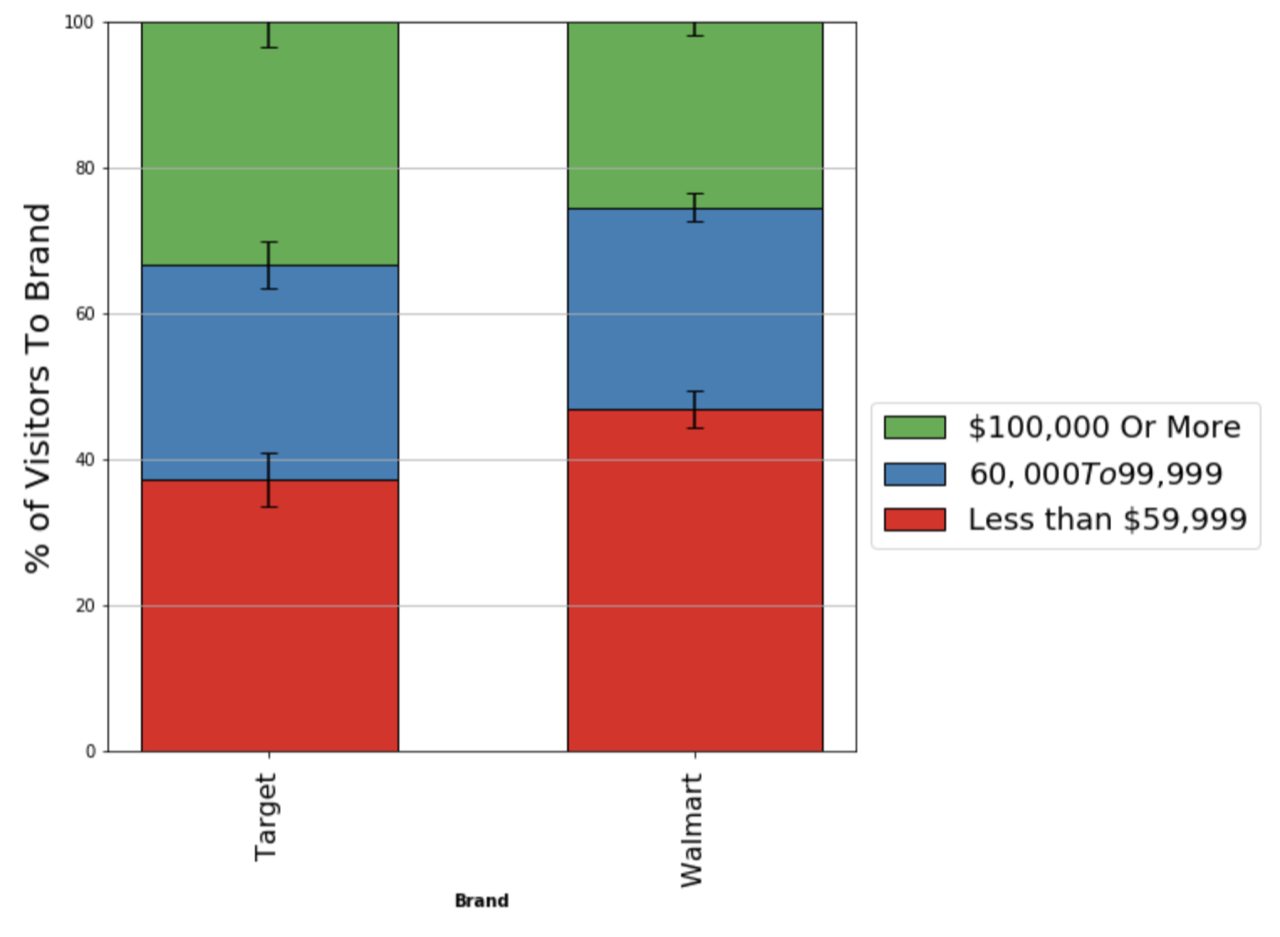Figure 4: Customer Demographics for a single location of Target vs a single location of Walmart. Error bars show 95% confidence intervals of the estimated mean rate of visitors from each segment.

Figure 4 is the same data as Figure 3, but now includes 95% confidence intervals of our estimates of each segment. Note the non-overlapping confidence intervals for the lowest income group (red bar) of Target vs Walmart. This is a statistically meaningful difference, and unlikely a result of random chance.

These results show that SafeGraph Patterns data can produce statistically meaningful demographic analysis of single POI locations, and reveal meaningful differences between two individual POI located in the same neighborhood.

## How exactly are these confidence intervals calculated?

If you want to understand exactly how these statistics are calculated, read on. As a reminder, the Teaching Notebook and Analysis Workbook implement and document this in python.

## Summary of Statistical Methodology

Here is a brief summary of our statistical methodology before we dive into the details.

1. We model each data point (each CBG visitor count) as a random draw from an independent Poisson distribution with some unknown rate parameter 𝜆. (Section 1)
2. When we sum together all individual data points (census block groups), the sum of Poissons is a new Poisson with mean and variance equal to the sum of the components. (Section 2)
3. We use the Normal approximation of a Poisson to estimate confidence intervals around the estimate of the mean. (Section 3)
4. We model each demographic segment independently and apply post-hoc stratified re-weighting (see Series Part 2) to correct our estimate of the mean (and its confidence interval) for each segment. Extrapolating widens our confidence intervals, but luckily the SafeGraph dataset is well sampled, so adjustments are minor. (Section 4)
5. We divide each estimate and confidence interval by total visitors to visualize data as a percent of total visitors. (See visualizations above and implementation in the Teacher Notebook)

## Section 1: We model each data point as a random draw from an independent Poisson distribution

To make statistical statements about our confidence or uncertainty, we need to decide how to model the data. Our demographic profile data is interesting because the individual measurements go through a few transformations and aggregations, which impacts the expected variance of the final measurement.

Let’s start at the beginning and walk through all of our data transformations to get to the demographic profile.

The raw data consists of measurements of many census_block_groups (CBGs) and many points-of-interest (POI)s (safegraph_place_ids).

For each CBG-POI pair, we have a count of visitors from that particular CBG_i to a particular POI_j, which we can model as a random draw from a Poisson distribution.

Where 𝜆ᵢ,ⱼ is the (unknown) rate parameter representing, on average, the count of visitors in the SafeGraph dataset with the home CBGᵢ will visit the particular POIⱼ in one month.

Then, we allocate this measurement of 𝑁 visitors into different 𝑘 demographic segments (e.g. Hispanic Or Latino Origin or Not Hispanic Or Latino Origin).

If we have 𝑁 visitors from CBGᵢ and, on average, 𝑝 fraction of them are Hispanic Or Latino Origin, then the count of Hispanic Or Latino Origin is a Binomial random variable. We know 𝑝 from the Census (i.e., what is the true fraction of Hispanic Or Latino Origin in CBGᵢ). So we can model this allocation as a binomial process where each visitor (of 𝑁ᵢ,ⱼ visitors) has a certain probability 𝑝 of being allocated to demographic segment 𝑘.

Where 𝐷𝑒𝑚𝑜𝑉𝑖𝑠𝑖𝑡𝑜𝑟𝑠ᵢ,ⱼ,𝑘 is the number of visitors from CBGᵢ visiting POIⱼ belonging to DemoSegment_k.

And 𝑝ᵢ,𝑘 is the fraction of residents in CBGᵢ belonging to DemoSegment_k (e.g., 0.15), which we know from the Census.

However, 𝐷𝑒𝑚𝑜𝑉𝑖𝑠𝑖𝑡𝑜𝑟𝑠 will not strictly behave as a true binomial random variable. That’s because its parameter ( 𝑁 ) is itself a random variable (not a fixed value) (this makes 𝐷𝑒𝑚𝑜𝑉𝑖𝑠𝑖𝑡𝑜𝑟𝑠 a compound distribution). Choosing this model is convenient because this particular compound distribution turns out itself to be mathematically equivalent to yet a new Poisson distribution (see stats.stackexchange for some discussion).

So to recap this logic:

𝐷𝑒𝑚𝑜𝑉𝑖𝑠𝑖𝑡𝑜𝑟𝑠 is our measurement of how many people of a specific demographic segment, from a specific CBG, visited a specific POI. The fact that we can model 𝐷𝑒𝑚𝑜𝑉𝑖𝑠𝑖𝑡𝑜𝑟𝑠 as a single Poisson distribution is extremely convenient because Poisson distributions are familiar statistical territory.

OK, we have a solid model for all of our individual data points. See “fine print” in the Teacher Notebook for a full accounting of our assumptions.

To get to our final results, we take three additional steps: (i) sum (ii) calculate confidence intervals (iii) correct bias.

## Section 2: A sum of Poissons is a Poisson

For each brand (e.g. Walmart) and each DemoSegment_k (e.g. Hispanic Or Latino Origin) we need to sum across all 𝐽 CBGs and 𝐼 POIs to get a total count of visitors from DemoSegment_k. Our motivating example is to compare a single location of Target and a single location of Walmart, so in that case 𝐼_𝑊𝑎𝑙𝑚𝑎𝑟𝑡 = 𝐼_𝑇𝑎𝑟𝑔𝑒𝑡=1, but alternatively we could analyze any number of locations in the USA. The actual number of stores in your analysis will depend on your question.

Since, each 𝐷𝑒𝑚𝑜𝑉𝑖𝑠𝑖𝑡𝑜𝑟𝑠_𝑖,𝑗,𝑘 is a Poisson random variable, then 𝐷𝑒𝑚𝑜𝑉𝑖𝑠𝑖𝑡𝑜𝑟𝑠_𝑘 is a sum of many Poisson random variables and itself a Poisson random variable. The sum of Poissons is a Poisson.

What this means is that even though we calculate 𝐷𝑒𝑚𝑜𝑉𝑖𝑠𝑖𝑡𝑜𝑟𝑠_𝑘 by summing over many 𝜆_𝑖,𝑗,𝑘 , for the purposes of quantifying uncertainty, 𝐷𝑒𝑚𝑜𝑉𝑖𝑠𝑖𝑡𝑜𝑟𝑠_𝑘 can be modeled as a random draw from a Poisson distribution with mean 𝜆_𝑘.

𝜆_𝑘 is the grand sum of all individual 𝜆_𝑖,𝑗,𝑘 (the individual rate parameters for visitors of CBGᵢ belonging to DemoSegment_k visiting POIⱼ).

Our estimate of 𝜆_𝑘 is the estimate for which we want to quantify our uncertainty. This is our estimate of how many people of DemoSegment_k visited this brand across all POI and all CBG.

## Section 3: Use the normal approximation to calculate confidence intervals

The whole point of this is that we want to quantify our uncertainty about our estimate of this Poisson rate 𝜆_𝑘 for each demographic segment 𝑘.

Although our individual CBG measurements may be small, aggregated together the sums are usually large (i.e. > 100) and we can comfortably use a normal approximation to approximate 𝑃𝑜𝑖𝑠𝑠𝑜𝑛(𝜆) as 𝑁𝑜𝑟𝑚𝑎𝑙(𝜆, sqrt(𝜆)). Methods to obtain confidence intervals of your estimate of 𝜆_𝑘 in such cases are well documented. For more details see stats.stackexchange and OnBiostatisics.

(And also, confidence intervals are confusing and controversial, so if you want to understand more about what confidence intervals mean, start here).

The takeaway is that we can calculate confidence intervals for a normal distribution with 𝜇=𝜆 and 𝜎=sqrt(𝜆). In our visualizations (e.g. see Figure 4) we show 95% confidence intervals around the estimate of the mean. If your sums are very small (i.e. < 100), and the normal approximation may not be reasonable, then you should consider calculating confidence intervals using the exact method. For an implementation in python see netwon.cx.

## Section 4: Correct geographic sampling bias via post-hoc stratified re-weighting

The last step is to correct for geographic sampling biases in our data collection. As discussed in the series Part 2, we know there may be some sampling bias in our data samples, and we need to apply post-hoc stratified re-weighting to correct for it.

In the function apply_strata_reweighting() we compute a re-weighting factor for every DemoSegment_k and multiply both the estimate of the mean and the confidence interval by this re-weighting factor. If SafeGraph is very under-indexed on a sub-group, then the re-weighting factor will be large, and, accordingly, it will expand our confidence intervals by a large amount. If you multiply a distribution by a constant 𝐶, then you multiply the variance of that distribution by 𝐶².

If you are paying attention, you may have noticed that we have done something a bit sneaky. When I explained how to do post-hoc stratified re-weighting in Series Part 2, I explained how to calculate the re-weighting adjustment factor for each individual CBG, based on the Census data, and then multiply the CBG visitor count by the re-weighting factor. Each CBG is its own group (i.e., stratum), and this is a geographic-based sampling correction. In other words, we apply the correction at the level of each individual CBG. But now I am showing that we apply re-weighting on each DemoSegment_k after summing together all of these CBGs. Why are we doing this differently than before? And how does it work?

Why is it different? Why do we correct sampling bias after summing instead of before?

The necessity of doing the correction after summing is for statistics. When you multiply a Poisson random variable by a constant (i.e. our adjust_factor), the result is no longer a Poisson random variable. If the individual measurements are not Poisson random variables, then their sum is no longer a Poisson random variable, which means we no longer know how to model the variance of their sum.

This is a problem because if we don’t know how to model the variance of the sum, then we don’t know how to build confidence intervals. The post-hoc stratified re-weighting correction expands our confidence intervals (we are extrapolating and that means less certainty); we can’t ignore this. If we want to know exactly how much our confidence intervals change, then we need a mathematically rigorous model of the variance of our measurements before and after the correction has been applied.

How does it work? How can we apply geographic-level corrections after we have summed all the geographies together?

Essentially, we first calculate our biases for each CBG as described in Part 2, and then we sum these biases together across CBGs, grouping them by each individual demographic segment. When we apply the correction after summing, we are technically correcting at the level of demographic segments, rather than the level of individual census block groups*. However, the demographic segments are just a linear transformation of the same data (we mapped from CBGs to Demographic segments via the Census), so they actually are two different views on the same dataset. In fact, whether you apply the correction on the individual CBG-level data before summing, or on the demographic segment after summing, it’s the same correction, and you get the same corrected estimate of the mean in either case. The only difference is that in the latter, you have a rigorous model of the variance of your estimate from which you can calculate confidence intervals. In the former, you don’t. If you want to see the calculation of the adjustment factor for a demographic segment or want to dig into how the math works, here is an example using a simple toy example.

* The adjustment factor for the demographic segment is essentially a ratio of the weighted sums of the corrected CBG-level counts for that segment :: weighted sums of uncorrected CBG-level counts for that segment.

## Section 5: Convert your estimates into percent-of-total for visualization.

When we finish Section 4, we now have k estimates, one for each 𝐷𝑒𝑚𝑜𝑉𝑖𝑠𝑖𝑡𝑜𝑟𝑠_k. For example, if we were analyzing Ethnicity, we would have two counts, one for Hispanic and one for Not Hispanic, representing our estimate as to how many people from each segment visited this brand (corrected for sampling bias). We also have confident estimates around each count.

To visualize these results, we normalize each count as a percent-of-total by dividing it by the sum of all k segments within its dimension. We also normalize the confidence intervals. This is not a statistical transformation that changes our statistical certainty, it is just changing the units of our estimate.Figure 4, reproduced. Customer Demographics for a single location of Target vs a single location of Walmart. Error bars show 95% confidence intervals of the estimated mean rate of visitors from each segment.

These are the final numbers and “error bars” that we use to build visualizations, like Figure 4.

## Summary of Statistical Methodology

Putting this all together, here is the summary of our statistical methodology, described in detail above.

1. We model each data point as a random draw from an independent Poisson distribution with some unknown rate parameter 𝜆. (Section 1)
2. When we sum together all individual data points (census block groups), the sum of Poissons is a new Poisson with mean and variance equal to the sum of the components. (Section 2)
3. We use the Normal approximation of a Poisson to estimate confidence intervals around the estimate of the mean. (Section 3)
4. We model each demographic segment independently and apply post-hoc stratified re-weighting to correct our estimate of the mean (and its confidence interval) for each segment. (Section 4)
5. We divide each estimate and confidence interval by total visitors to visualize data as a percent of total visitors. (See visualizations above and implementation in the Teacher Notebook)

## You are ready to take demographic profiles to the next level

Thank you for reading! We’d love to hear from you and hear about what research or business questions you are working on. Drop us a line at [email protected]. If you haven’t read all 4 parts, here are links to the other members of the series.

Thanks for reading! If you found this useful or interesting please upvote and share with a friend. You are strongly encouraged to try out a sample of SafeGraph patterns data for free, no strings attached at the SafeGraph Data Bar. Use coupon code AnalyzeDemographics for \$200 worth of free data! Also, please send us your ideas, feedback, bug discoveries and suggestions: [email protected]

## Browse the latest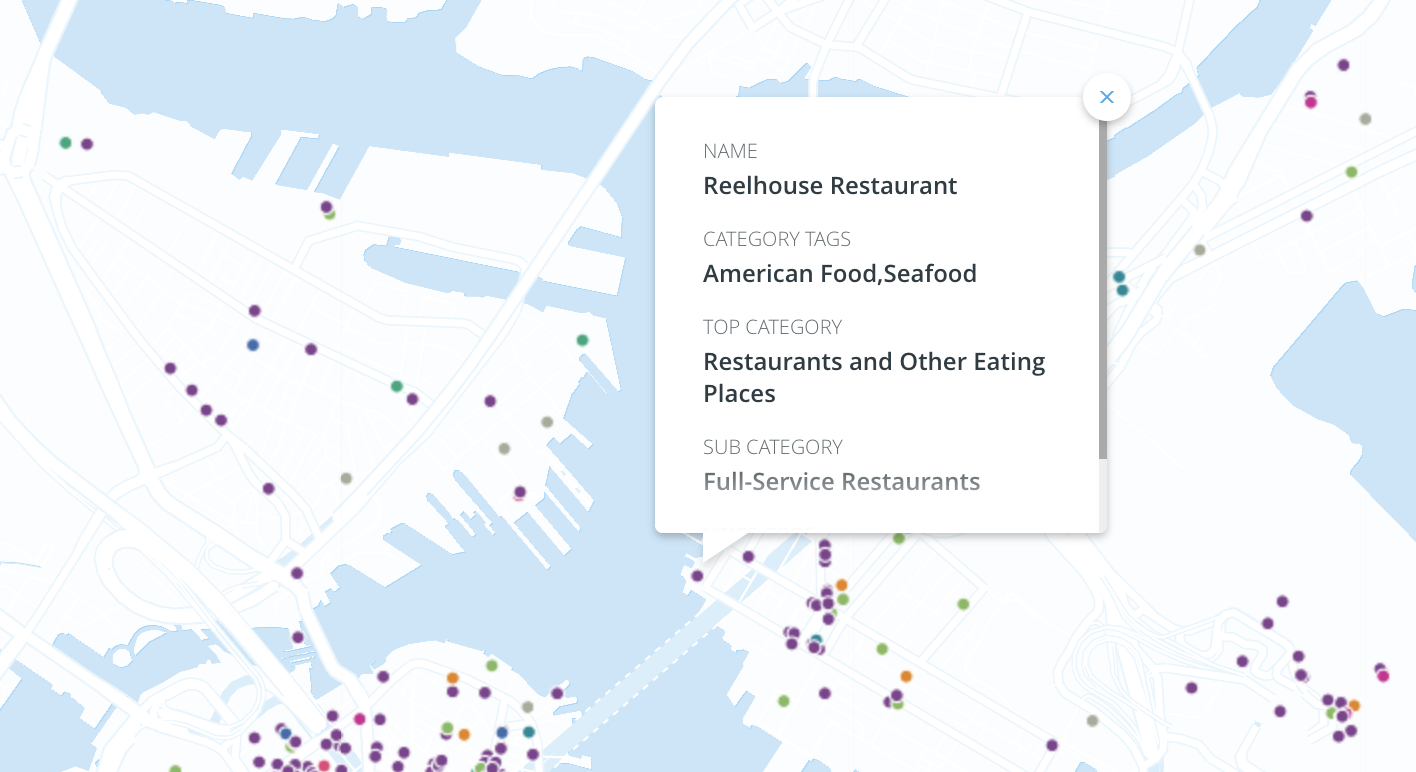Demystifying the SafeGraph Facts
May 3, 2022
by
Auren Hoffman